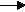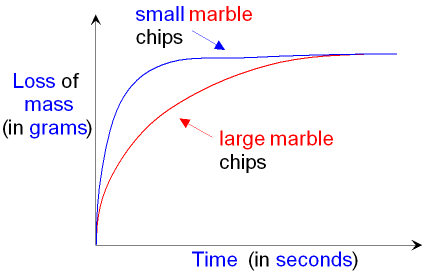gcsescience.com                                       10                                       gcsescience.com

Rates of Reaction

What is the Effect of Increasing the Surface Area
of a Solid on the Rate of a Chemical Reaction?

A solid that is present in a solution can only react
when particles collide with the surface of the solid.
If the area of the solid surface is made bigger
then more particles can collide with it per second
and the rate of the chemical reaction increases.

You can increase the surface area of a solid by breaking
A solid that is broken down into a powder has the largest
surface area and therefore has the fastest reaction rate.
This is why a catalyst is often used in the form of a powder.

In the reaction between calcium carbonate
and dilute hydrochloric acid, calcium carbonate
may be used in the form of marble chips.

HCl + calcium carbonatecalcium chloride + carbon dioxide + water.
2HCl(aq)   +   CaCO3(s)CaCl2(aq)     +      CO2(g)   +    H2O(l)

The reaction rates of both large marble chips and
small marble chips can be compared - see below.
A fair test must use the same mass of marble chips
for each reaction. The reaction rate can be
followed by plotting the loss of mass against time.The reaction rate is faster (the slope is steeper)
for the reaction with the small marble chips because
these have a greater surface area and
therefore they produce carbon dioxide more quickly.

Both lines on the graph finish at the same place. This
shows that the final loss of mass (the amount of carbon
dioxide
produced) is the same for both reactions.
This is because the same mass of calcium carbonate
(marble chips) will produce the same mass of
carbon dioxide whether the chips are large or small.
The smaller chips will just do it more quickly.

gcsescience.com      The Periodic Table      Index      Reaction Rate Quiz      gcsescience.com